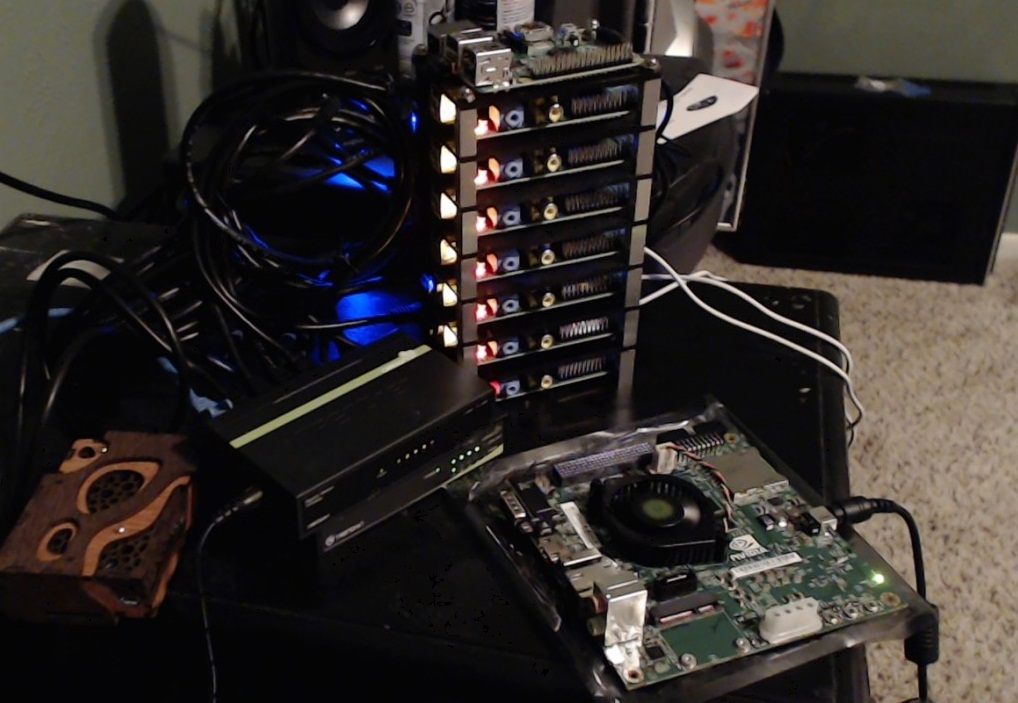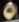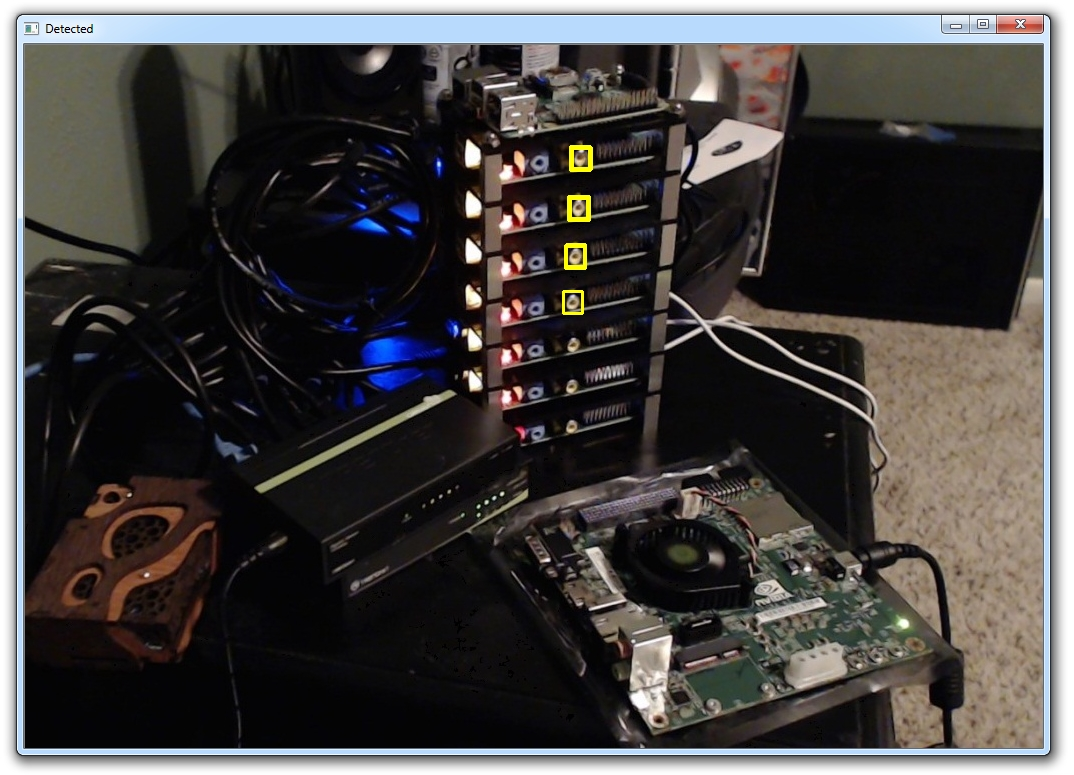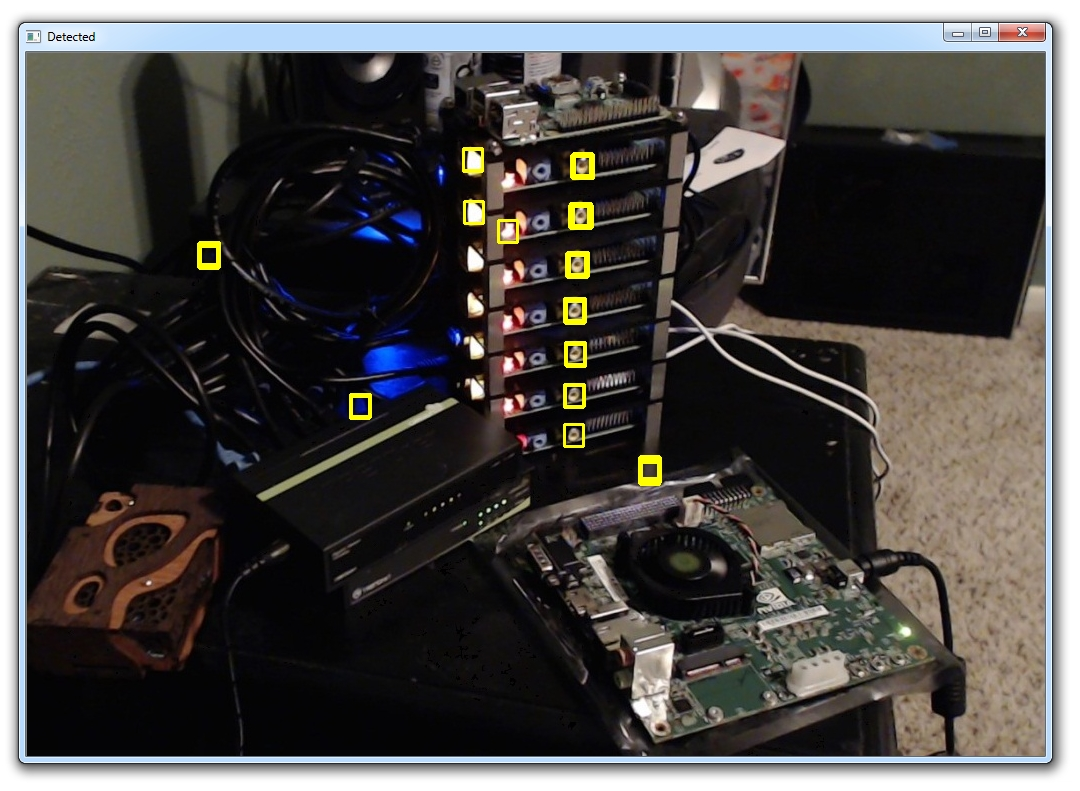## Template Matching OpenCV Python Tutorial

Welcome to another OpenCV with Python tutorial, in this tutorial we're going to cover a fairly basic version of object recognition. The idea here is to find identical regions of an image that match a template we provide, giving a certain threshold. For exact object matches, with exact lighting/scale/angle, this can work great. An example where these conditions are usually met is just about any GUI on the computer. The buttons and such are always the same, so you can use template matching. Pair template matching with some mouse controls and you've got yourself a web-based bot!

To start, you will need a main image, and a template. You should take your template from the exact "thing" you are looking for in the image. I will provide an image as an example, but feel free to use an image of your favorite website or something like that.

Main image:Template that we will search for: (ports on the Pis)This is just one of the ports, but we're curious to see if we can match any of the others. We do have a threshold option, where if something is maybe an 80% match, then we say it's a match. So, we will start with loading in and converting the images

```import cv2
import numpy as np

img_gray = cv2.cvtColor(img_rgb, cv2.COLOR_BGR2GRAY)

w, h = template.shape[::-1]```

Thus far, we load in both images, convert to gray. We keep the original RGB image, and create a grayscale version. I've mentioned this before, but the reason why we do this is because we do all of the processing in the grayscale version, then use the same coordinates for labels and such on the color image.

With the main image, we just have the color version and the grayscale version. We load the template and note the dimensions.

```res = cv2.matchTemplate(img_gray,template,cv2.TM_CCOEFF_NORMED)
threshold = 0.8
loc = np.where( res >= threshold)```

Here, we call res the matchTemplate between the img_gray (our main image), the template, and then the matching method we're going to use. We specify a threshold, here 0.8 for 80%. Then we find locations with a logical statement, where the res is greater than or equal to 80%.

Finally, we mark all matches on the original image, using the coordinates we found in the gray image:

```for pt in zip(*loc[::-1]):
cv2.rectangle(img_rgb, pt, (pt + w, pt + h), (0,255,255), 2)

cv2.imshow('Detected',img_rgb)```So we got a few matches. Maybe lower the threshold? We'll try `threshold = 0.7`.Got some false positives here. You could continue adjusting the threshold until you had 100%, but you may never get there without false positives. Another option would be to just take another template image. Sometimes, it can be useful to have multiple images of the same object. This way, you can keep your threshold high enough to be relatively certain that your results will be accurate.

In the next tutorial, we're going to cover foreground extraction.

The next tutorial:• Drawing and Writing on Image OpenCV Python Tutorial

• Image Operations OpenCV Python Tutorial

• Image arithmetics and Logic OpenCV Python Tutorial

• Thresholding OpenCV Python Tutorial

• Color Filtering OpenCV Python Tutorial

• Blurring and Smoothing OpenCV Python Tutorial

• Morphological Transformations OpenCV Python Tutorial

• Canny Edge Detection and Gradients OpenCV Python Tutorial

• Template Matching OpenCV Python Tutorial
• GrabCut Foreground Extraction OpenCV Python Tutorial

• Corner Detection OpenCV Python Tutorial

• Feature Matching (Homography) Brute Force OpenCV Python Tutorial

• MOG Background Reduction OpenCV Python Tutorial

• Haar Cascade Object Detection Face & Eye OpenCV Python Tutorial